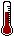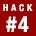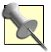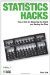# Hack 4. Reject the NullExperimental scientists make progress by making a guess that they are sure is wrong.

Science is a goal-driven process, and the goal is to build a body of knowledge about the world. The body of knowledge is structured as a long list of scientific laws, rules, and theories about how things work and how they are. Experimental science introduces new laws and theories and tests them through a logical set of steps known as hypothesis testing.

#### Hypothesis Testing

A hypothesis is a guess about the world that is testable. For example, I might hypothesize that washing my car causes it to rain or that getting into a bathtub causes the phone to ring. In these hypotheses, I am suggesting a relationship between car washing and rainfall or between bathing and phone calls.

A reasonable way to see whether these hypotheses are true is to make observations of the variables in the hypothesis (for the sake of sounding like statisticians, we'll call that collecting data) and see whether a relationship is apparent. If the data suggests there is a relationship between my variables of interest, my hypothesis is supported, and I might reasonably continue to believe my guess is correct. If no relationship is apparent in the data, then I might wisely begin to doubt that my hypothesis is true or even reject it altogether.

There are four possible outcomes when scientists test hypotheses by collecting data. Table 1-3 shows the possible outcomes for this decision-making process.

##### Table Possible outcomes of research hypothesis testing
Hypothesis is correct: the world really is this way Hypothesis is wrong: the world really is not this way
Data does support hypothesis: accept hypothesisA. Correct decision: science makes progress.B. Wrong decision: science is thwarted!
Data does not support hypothesis: reject hypothesisC. Wrong decision: drat, foiled again!D. Correct decision:science makes progress.

Outcomes A and D add to science's body of knowledge. Though A is more likely to make a research scientist all wriggly, D is just fine. Outcomes B and C, though, are mistakes, and represent misinformation that only confuses our understanding of the world.

#### Statistical Hypothesis Testing

The process of hypothesis testing probably makes sense to youit is a fairly intuitive way to reach conclusions about the world and the people in it. People informally do this sort of hypothesis testing all the time to make sense of things.

Statisticians also test hypotheses, but hypotheses of a very specific variety. First, they have data that represents a sample of values from a real or theoretical population about which they wish to reach conclusions. So, their hypotheses are about populations. Second, they usually have hypotheses about the existence of a relationship among variables in the population of interest. A generic statistician's research hypothesis looks like this: there is a relationship between variable X and variable Y in the population of interest.

Unlike research hypothesis testing, with statistical hypothesis testing, the probability statement that a statistician makes at the end of the hypothesis testing process is not related to the likelihood that the research hypothesis is true. Statisticians produce probability statements about the likelihood that the research hypothesis is false. To be more technically accurate, statisticians make a statement about whether a hypothesis opposite to the research hypothesis is likely to be correct. This opposite hypothesis is typically a hypothesis of no relationship among variables, and is called the null hypothesis. A generic statistician's null hypothesis looks like this: there is no relationship between variable X and variable Y in the population of interest.

The research and null hypotheses cover all the bases. There either is or is not a relationship among variables. Essentially, when having to choose between these two hypotheses, concluding that one is false provides support for the other. Logically, then, this approach is just as sound as the more intuitive approach presented earlier and utilized naturally by humans every day. The preferred outcome by researchers conducting null hypothesis testing is a bit different than the general hypothesis-testing approach presented in Table 1-3.

As Table 1-4 shows, statisticians usually wish to reject their hypothesis. It is by rejecting the null that statistical researchers confirm their research hypotheses, get the grants, receive the Nobel prize, and one day are rewarded with their faces on a postage stamp.

##### Table Possible outcomes of null hypothesis testing
Null hypothesis is correct:there is no relationship in the population Null hypothesis is wrong: there is a relationship in the population
Data does support null hypothesis: fail to reject the nullA. Correct decision: science makes progress.B. Wrong decision: science is thwarted!
Data does not support null hypothesis: reject the null C. Wrong decision: drat, foiled again!D. Correct decision:science makes progress.

Although outcome A is still OK (as far as science is concerned), it is now outcome D that pleases researchers because it indicates support for their real guesses about the world, their research hypotheses. Outcomes B and C are still mistakes that hamper scientific progress.

#### Why It Works

Statisticians test the null hypothesisguess the opposite of what they hope to findfor several reasons. First, proving something to be true is really, really tough, especially if the hypothesis involves a specific value, as statistical research often does. It is much easier to prove that a precise guess is wrong than prove that a precise guess is true. I can't prove that I am 29 years old, but it would be pretty easy to prove I am not.

It is also comparatively easy to show that any particular estimate of a population value is not likely to be correct. Most null hypotheses in statistics suggest that a population value is zero (i.e., there is no relationship between X and Y in the population of interest), and all it takes to reject the null is to argue that whatever the population value is, it probably isn't zero. Support for researchers' hypotheses generally come by simply demonstrating that the population value is greater than nothing, without specifically saying what that population value is exactly.Quite a perk for the professional statistician, eh? All the statistician has to do is tell you that your answer is wrong, not tell you what the right answer is!

Even without using numbers as an example, philosophers of science have long argued that progress is best made in science by postulating hypotheses and then attempting to prove that they are wrong. For good science, falsifiable hypotheses are the best kind.

It is the custom to conduct statistical analyses this way: present a null hypothesis that is the opposite of the research hypothesis and see whether you can reject the null. R.A. Fisher, the early 20th century's greatest statistician, suggested this approach, and it has stuck. There are other methods, though. Plenty of modern statisticians have argued that we should concentrate on producing the best estimate of those population values of interest (such as the size of relationships among variables), instead of focusing on proving that the relationship is the size of some nonspecified number not equal to zero.Statistics Hacks: Tips & Tools for Measuring the World and Beating the Odds
ISBN: 0596101643
EAN: 2147483647
Year: 2004
Pages: 114
Authors: Bruce Frey

Similar book on Amazon